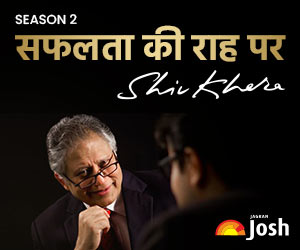# NCERT Solutions for Class 12 Physics Chapter 14 Semiconductor Electronics Material Devices and Simple Circuits, PDF Download

NCERT Solutions for Class 12 Physics Chapter 14 Semiconductor Electronics Material Devices and Simple Circuits: Get here the NCERT Class 12 Semiconductor Electronics Material Devices and Simple Circuits Solutions in PDF format. The solutions follow the revised NCERT Class 12 Physics textbook.NCERT Solutions for Class 12 Physics Chapter 14 Semiconductor Electronics Material Devices and Simple Circuits

NCERT Class 12 Physics Solutions: Students of Class 12 can refer to this article to get NCERT Solutions for Class 12 Physics Chapter 14. These solutions will be helpful in understanding Chapter 14 Semiconductor Electronics Material Devices and Simple Circuits. The NCERT Solutions for Class 12 Chapter 14 Semiconductor Electronics Material Devices and Simple Circuits will include all unsolved theories and numerical problems. Read to get NCERT solutions for Class 12 Physics, Chapter 14 all exercises.

Get, detailed solutions to the questions of the chapter Semi Conductor Electronics Material Devices and Simple Circuit from NCERT textbooks. The objective is to helping students regarding the pattern of answering the question as per the cbse latest marking scheme.## Semiconductor Electronics Material Devices and Simple Circuits Solutions

Some questions of this chapter are given here.

Q. In an n-type silicon, which of the following statement is true:
a) Electrons are majority carriers and trivalent atoms are the dopants.
b) Electrons are minority carriers and pentavalent atoms are the dopants.
c) Holes are minority carriers and pentavalent atoms are the dopants.
d) Holes are majority carriers and trivalent atoms are the dopants.

Sol.  (c)

Q. Which of the statements given in Exercise 14.1 is true for p-type semiconductors.

Sol. (d)

Q. Carbon, silicon and germanium have four valence electrons each. These are characterised by valence and conduction bands separated by energy band gap respectively equal to (Eg)C, (Eg)Si and (Eg)Ge. Which of the following statements is true?
a) (Eg)Si < (Eg)Ge< (Eg)C
b) (Eg)C < (Eg)Ge> (Eg)Si
c) (Eg)C > (Eg)Si > (Eg)Ge
d) (Eg)C = (Eg)Si = (Eg)Ge

Sol. (c)

Q. For transistor action, which of the following statements are correct:
a) Base, emitter and collector regions should have similar size and doping concentrations.
b) The base region must be very thin and lightly doped.
c) The emitter junction is forward biased and collector junction is reverse biased.
d) Both the emitter junction as well as the collector junction is forward biased.

Sol. (b), (c)

Q.
In an unbiased p-n junction, holes diffuse from the p-region ton-region because
a) free electrons in the n-region attract them.
b) they move across the junction by the potential difference.
c) hole concentration in p-region is more as compared to n-region.
d) All the above.

Sol. (c)

Q. When a forward bias is applied to a p-n junction, it
a) Raises the potential barrier.
b) Reduces the majority carrier current to zero.
c) Lowers the potential barrier.
d) None of the above.

Sol. (c)

The answers to these questions and many more are given in the PDF attached in the below link. Click to see the answers.

## NCERT Rationalised Content Chapter: Semiconductor Electronics Material Devices and Simple Circuits

 Chapter Name Page No. Deleted Topics Chapter 14: Semiconductor Electronics: Material Devices and Simple Circuits 485–495 14.8 Special Purpose p-n junction Diodes 14.9 Digital Electronics and Logic Gates     Exercises 14.7–14.15 497–499

## CBSE Class 12 Physics Updated Question Paper Design 2023-24

 Typology of Questions Total Marks Approximate % Remembering: Exhibit memory of previously learned material by recalling facts, terms, basic concepts, and answers.  Understanding: Demonstrate understanding of facts and ideas by organising, comparing, translating, interpreting, giving descriptions, and stating main ideas. 27 38 Applying: Solve problems to new situations by applying acquired knowledge, facts, techniques and rules in a different way. 22 32 Analysing: Examine and break information into parts by identifying motives or causes. Make inferences and find evidence to support generalisations.  Evaluating: Present and defend opinions by making judgments about information, validity of ideas, or quality of work based on a set of criteria.  Creating: Compile information together in a different way by combining elements in a new pattern or proposing alternative solutions. 21 30 Total 70 100 Practical 30 Gross Total 100

Also Check:

## Related Categories

खेलें हर किस्म के रोमांच से भरपूर गेम्स सिर्फ़ जागरण प्ले पर